# NCERT solutions for Mathematics Exemplar Class 10 chapter 6 - Triangles [Latest edition]

#### Chapters## Chapter 6: Triangles

Exercise 6.1Exercise 6.2Exercise 6.3Exercise 6.4
Exercise 6.1 [Pages 60 - 62]

### NCERT solutions for Mathematics Exemplar Class 10 Chapter 6 Triangles Exercise 6.1 [Pages 60 - 62]

#### Choose the correct alternative:

Exercise 6.1 | Q 1 | Page 60

In Figure, ∠BAC = 90° and AD ⊥ BC. Then, ______.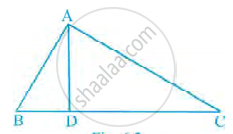• BD . CD = BC2

• AB . AC = BC2

• BD . CD = AD2

• AB . AC = AD2

Exercise 6.1 | Q 2 | Page 61

The lengths of the diagonals of a rhombus are 16 cm and 12 cm. Then, the length of the side of the rhombus is ______.

• 9 cm

• 10 cm

• 8 cm

• 20 cm

Exercise 6.1 | Q 3 | Page 61

If ΔABC ~ ΔEDF and ΔABC is not similar to ΔDEF, then which of the following is not true?

• BC . EF = A C. FD

• AB . EF = AC . DE

• BC . DE = AB . EF

• BC . DE = AB . FD

Exercise 6.1 | Q 4 | Page 61

If in two triangles ABC and PQR, (AB)/(QR) = (BC)/(PR) = (CA)/(PQ), then ______.

• ΔPQR ~ ΔCAB

• ΔPQR ~ ΔABC

• ΔCBA ~ ΔPQR

• ΔBCA ~ ΔPQR

Exercise 6.1 | Q 5 | Page 61

In figure, two line segments AC and BD intersect each other at the point P such that PA = 6 cm, PB = 3 cm, PC = 2.5 cm, PD = 5 cm, ∠APB = 50° and ∠CDP = 30°. Then, ∠PBA is equal to ______.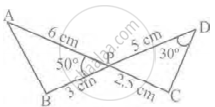• 50°

• 30°

• 60°

• 100°

Exercise 6.1 | Q 6 | Page 61

If in two triangles DEF and PQR, ∠D = ∠Q and ∠R = ∠E, then which of the following is not true?

• (EF)/(PR) = (DF)/(PQ)

• (DE)/(PQ) = (EF)/(RP)

• (DE)/(QR) = (DF)/(PQ)

• (EF)/(RP) = (DE)/(QR)

Exercise 6.1 | Q 7 | Page 62

In triangles ABC and DEF, ∠B = ∠E, ∠F = ∠C and AB = 3DE. Then, the two triangles are ______.

• congruent but not similar

• similar but not congruent

• neither congruent nor similar

• congruent as well as similar

Exercise 6.1 | Q 8 | Page 62

It is given that ΔABC ~ ΔPQR, with (BC)/(QR) = 1/3. Then, (ar(PRQ))/(ar(BCA)) is equal to ______.

• 9

• 3

• 1/3

• 1/9

Exercise 6.1 | Q 9 | Page 62

It is given that ΔABC ~ ΔDFE, ∠A =30°, ∠C = 50°, AB = 5 cm, AC = 8 cm and DF = 7.5 cm. Then, the following is true ______.

• DE = 12 cm, ∠F = 50°

• DE = 12 cm, ∠F = 100°

• EF = 12 cm, ∠D = 100°

• EF = 12 cm, ∠D = 30°

Exercise 6.1 | Q 10 | Page 62

If in triangles ABC and DEF, (AB)/(DE) = (BC)/(FD), then they will be similar, when ______.

• ∠B = ∠E

• ∠A = ∠D

• ∠B = ∠D

• ∠A = ∠F

Exercise 6.1 | Q 11 | Page 62

If ∆ABC ~ ∆QRP, (ar(ABC))/(ar(PQR)) = 9/4, AB = 18 cm and BC = 15 cm, then PR is equal to ______.

• 10 cm

• 12 cm

• 20/3 cm

• 8 cm

Exercise 6.1 | Q 12 | Page 62

If S is a point on side PQ of a ΔQR such that PS = QS = RS, then ______.

• PR . QR = RS2

• QS2 + RS2 = QR2

• PR2 + QR2 = PQ2

• PS2 + RS2 = PR2

Exercise 6.2 [Pages 63 - 65]

### NCERT solutions for Mathematics Exemplar Class 10 Chapter 6 Triangles Exercise 6.2 [Pages 63 - 65]

Exercise 6.2 | Q 1 | Page 63

Is the triangle with sides 25 cm, 5 cm and 24 cm a right triangle? Give reasons for your answer.

Exercise 6.2 | Q 2 | Page 63

It is given that ΔDEF ~ ΔRPQ. Is it true to say that ∠D = ∠R and ∠F = ∠P? Why?

Exercise 6.2 | Q 3 | Page 63

A and B are respectively the points on the sides PQ and PR of a triangle PQR such that PQ = 12.5 cm, PA = 5 cm, BR = 6 cm and PB = 4 cm. Is AB || QR? Give reasons for your answer.

Exercise 6.2 | Q 4 | Page 64

In figure, BD and CE intersect each other at the point P. Is ΔPBC ~ ΔPDE? Why?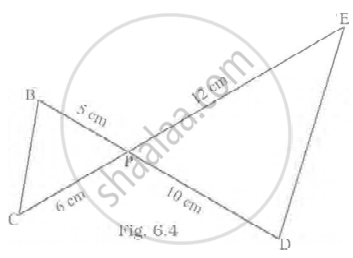Exercise 6.2 | Q 5 | Page 64

In ∆PQR and ∆MST, ∠P = 55°, ∠Q = 25°, ∠M = 100° and ∠S = 25°. Is ∆QPR ~ ∆TSM? Why?

Exercise 6.2 | Q 6 | Page 64

Is the following statement true? Why? “Two quadrilaterals are similar, if their corresponding angles are equal”.

• True

• False

Exercise 6.2 | Q 7 | Page 64

Two sides and the perimeter of one triangle are respectively three times the corresponding sides and the perimeter of the other triangle. Are the two triangles similar? Why?

Exercise 6.2 | Q 8 | Page 129

Using Converse of basic proportionality theorem, prove that the line joining the mid-points of any two sides of a triangle is parallel to the third side. (Recall that you have done it in Class IX).

Exercise 6.2 | Q 9 | Page 64

The ratio of the corresponding altitudes of two similar triangles is 3/5. Is it correct to say that ratio of their areas is 6/5? Why?

Exercise 6.2 | Q 10 | Page 65

D is a point on side QR of ΔPQR such that PD ⊥ QR. Will it be correct to say that ΔPQD ~ ΔRPD? Why?

Exercise 6.2 | Q 11 | Page 65

In figure, if ∠D = ∠C, then it is true that ΔADE ~ ΔACB? Why?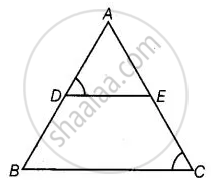Exercise 6.2 | Q 12 | Page 65

Is it true to say that if in two triangles, an angle of one triangle is equal to an angle of another triangle and two sides of one triangle are proportional to the two sides of the other triangle, then the triangles are similar? Give reasons for your answer.

Exercise 6.3 [Pages 66 - 69]

### NCERT solutions for Mathematics Exemplar Class 10 Chapter 6 Triangles Exercise 6.3 [Pages 66 - 69]

Exercise 6.3 | Q 1 | Page 66

In a ∆PQR, PR2 – PQ2 = QR2 and M is a point on side PR such that QM ⊥ PR. Prove that QM2 = PM × MR.

Exercise 6.3 | Q 2 | Page 67

Find the value of x for which DE || AB in figure.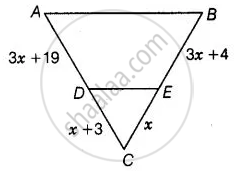Exercise 6.3 | Q 3 | Page 67

In figure, if ∠1 =∠2 and ΔNSQ ≅ ΔMTR, then prove that ΔPTS ~ ΔPRQ.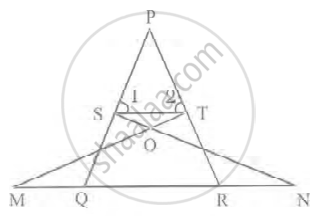Exercise 6.3 | Q 4 | Page 140

In the figure (QR)/(QS) = (QT)/(PR) and ∠1 = ∠2. Show that ∆PQS ~ ∆TQR.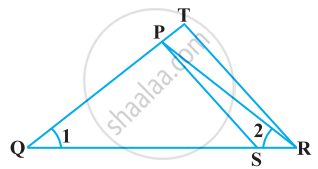Exercise 6.3 | Q 5 | Page 67

In figure, if AB || DC and AC, PQ intersect each other at the point O. Prove that OA . CQ = OC . AP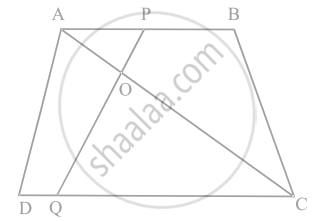Exercise 6.3 | Q 6 | Page 68

Find the altitude of an equilateral triangle of side 8 cm.

Exercise 6.3 | Q 7 | Page 68

If ∆ABC ~ ∆DEF, AB = 4 cm, DE = 6 cm, EF = 9 cm and FD = 12 cm, find the perimeter of ∆ABC.

Exercise 6.3 | Q 8 | Page 68

In figure, if DE || BC, find the ratio of ar(ΔADE) and ar (DECB).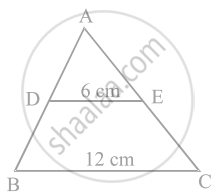Exercise 6.3 | Q 9 | Page 68

ABCD is a trapezium in which AB || DC and P and Q are points on AD and BC, respectively such that PQ || DC. If PD = 18 cm, BQ = 35 cm and QC = 15 cm, find AD.

Exercise 6.3 | Q 10 | Page 68

Corresponding sides of two similar triangles are in the ratio of 2 : 3. If the area of the smaller triangle is 48 cm2, find the area of the larger triangle.

Exercise 6.3 | Q 11 | Page 68

In a triangle ∆PQR, N is a point on PR such that QN ⊥ PR. If PN. NR = QN2, prove that ∠PQR = 90°.

Exercise 6.3 | Q 12 | Page 69

Areas of two similar triangles are 36 cm2 and 100 cm2. If the length of a side of the larger triangle is 20 cm, find the length of the corresponding side of the smaller triangle.

Exercise 6.3 | Q 13 | Page 69

In the figure, if ∠ACB = ∠CDA, AC = 8 cm and AD = 3 cm, find BD.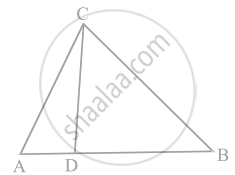Exercise 6.3 | Q 14 | Page 69

A 15 metres high tower casts a shadow 24 metres long at a certain time and at the same time, a telephone pole casts a shadow 16 metres long. Find the height of the telephone pole.

Exercise 6.3 | Q 15 | Page 69

Foot of a 10 m long ladder leaning against a vertical wall is 6 m away from the base of the wall. Find the height of the point on the wall where the top of the ladder reaches.

Exercise 6.4 [Pages 73 - 76]

### NCERT solutions for Mathematics Exemplar Class 10 Chapter 6 Triangles Exercise 6.4 [Pages 73 - 76]

Exercise 6.4 | Q 1 | Page 73

In figure, if ∠A = ∠C, AB = 6 cm, BP = 15 cm, AP = 12 cm and CP = 4 cm, then find the lengths of PD and CD.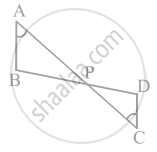Exercise 6.4 | Q 2 | Page 73

It is given that ∆ABC ~ ∆EDF such that AB = 5 cm, AC = 7 cm, DF = 15 cm and DE = 12 cm. Find the lengths of the remaining sides of the triangles.

Exercise 6.4 | Q 3 | Page 73

Prove that if a line is drawn parallel to one side of a triangle to intersect the other two sides, then the two sides are divided in the same ratio.

Exercise 6.4 | Q 4 | Page 73

In figure, if PQRS is a parallelogram and AB || PS, then prove that OC || SR.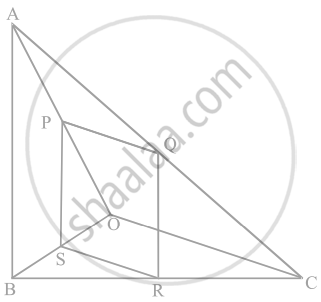Exercise 6.4 | Q 5 | Page 73

A 5 m long ladder is placed leaning towards a vertical wall such that it reaches the wall at a point 4 m high. If the foot of the ladder is moved 1.6 m towards the wall, then find the distance by which the top of the ladder would slide upwards on the wall.

Exercise 6.4 | Q 6 | Page 73

For going to a city B from city A, there is a route via city C such that AC ⊥ CB, AC = 2x km and CB = 2(x + 7) km. It is proposed to construct a 26 km highway which directly connects the two cities A and B. Find how much distance will be saved in reaching city B from city A after the construction of the highway.

Exercise 6.4 | Q 7 | Page 74

A flag pole 18 m high casts a shadow 9.6 m long. Find the distance of the top of the pole from the far end of the shadow.

#### Tick the correct answer and justify:

Exercise 6.4 | Q 8 | Page 74

A street light bulb is fixed on a pole 6 m above the level of the street. If a woman of height 1.5 m casts a shadow of 3 m, find how far she is away from the base of the pole.

Exercise 6.4 | Q 9 | Page 74

In figure, ABC is a triangle right angled at B and BD ⊥ AC. If AD = 4 cm, and CD = 5 cm, find BD and AB.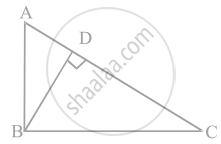Exercise 6.4 | Q 10 | Page 74

In figure, PQR is a right triangle right angled at Q and QS ⊥ PR. If PQ = 6 cm and PS = 4 cm, find QS, RS and QR.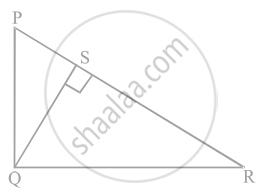Exercise 6.4 | Q 11 | Page 74

In ∆PQR, PD ⊥ QR such that D lies on QR. If PQ = a, PR = b, QD = c and DR = d, prove that (a + b)(a – b) = (c + d)(c – d).

Exercise 6.4 | Q 12 | Page 74

In a quadrilateral ABCD, ∠A + ∠D = 90°. Prove that AC2 + BD2 = AD2 + BC2

Exercise 6.4 | Q 13 | Page 75

In figure, l || m and line segments AB, CD and EF are concurrent at point P. Prove that (AE)/(BF) = (AC)/(BD) = (CE)/(FD).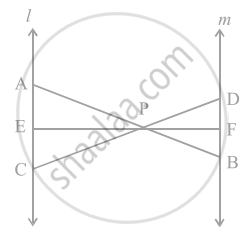Exercise 6.4 | Q 14 | Page 75

In figure, PA, QB, RC and SD are all perpendiculars to a line l, AB = 6 cm, BC = 9 cm, CD = 12 cm and SP = 36 cm. Find PQ, QR and RS.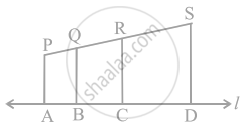Exercise 6.4 | Q 15 | Page 75

O is the point of intersection of the diagonals AC and BD of a trapezium ABCD with AB || DC. Through O, a line segment PQ is drawn parallel to AB meeting AD in P and BC in Q. Prove that PO = QO.

Exercise 6.4 | Q 16 | Page 75

In figure, line segment DF intersect the side AC of a triangle ABC at the point E such that E is the mid-point of CA and ∠AEF = ∠AFE. Prove that (BD)/(CD) = (BF)/(CE).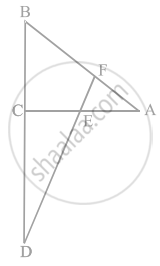Exercise 6.4 | Q 17 | Page 76

Prove that the area of the semicircle drawn on the hypotenuse of a right-angled triangle is equal to the sum of the areas of the semicircles drawn on the other two sides of the triangle.

Exercise 6.4 | Q 18 | Page 76

Prove that the area of the equilateral triangle drawn on the hypotenuse of a right-angled triangle is equal to the sum of the areas of the equilateral triangles drawn on the other two sides of the triangle.

## Chapter 6: Triangles

Exercise 6.1Exercise 6.2Exercise 6.3Exercise 6.4## NCERT solutions for Mathematics Exemplar Class 10 chapter 6 - Triangles

NCERT solutions for Mathematics Exemplar Class 10 chapter 6 (Triangles) include all questions with solution and detail explanation. This will clear students doubts about any question and improve application skills while preparing for board exams. The detailed, step-by-step solutions will help you understand the concepts better and clear your confusions, if any. Shaalaa.com has the CBSE Mathematics Exemplar Class 10 solutions in a manner that help students grasp basic concepts better and faster.

Further, we at Shaalaa.com provide such solutions so that students can prepare for written exams. NCERT textbook solutions can be a core help for self-study and acts as a perfect self-help guidance for students.

Concepts covered in Mathematics Exemplar Class 10 chapter 6 Triangles are Similar Figures, Similarity of Triangles, Basic Proportionality Theorem (Thales Theorem), Criteria for Similarity of Triangles, Areas of Similar Triangles, Application of Pythagoras Theorem in Acute Angle and Obtuse Angle, Triangles Examples and Solutions, Angle Bisector, Ratio of Sides of Triangle, Right-angled Triangles and Pythagoras Property, Similarity of Triangles, Similarity of Triangles.

Using NCERT Class 10 solutions Triangles exercise by students are an easy way to prepare for the exams, as they involve solutions arranged chapter-wise also page wise. The questions involved in NCERT Solutions are important questions that can be asked in the final exam. Maximum students of CBSE Class 10 prefer NCERT Textbook Solutions to score more in exam.

Get the free view of chapter 6 Triangles Class 10 extra questions for Mathematics Exemplar Class 10 and can use Shaalaa.com to keep it handy for your exam preparation